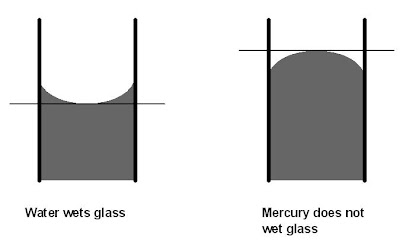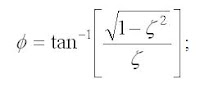Custom Search

## Thursday, March 7, 2013

### “U”-tube liquid filled manometers

These manometers are used for measurement of gauge pressures (up to 0.1 MPa), vacuumetric pressures (down to 0.1 MPa below atmospheric pressure) and for differential pressures of liquids and gases. The principle is based on the static balance between the measured pressure and the head of the liquid column. Fig. 1 shows the schematic of this manometer. A glass tube 1, bended to the “U”-shape, is filled by one half of its volume with liquid 2 (water, mercury). This tube is placed vertically, and a scale 3 (usually in mm) is attached along its height. Pressures P1 and P2 are supplied to legs of the tube, and levels of liquid in the legs change their position.When static balance between a measuring pressure and the head of the liquid column is reached, this pressure can be evaluated according to the equation:

where,

P1 and  P2        - pressures supplied to the legs of the manometer,

Pa;h1 and h2          - deviations of liquid levels from the zero point of the scale in two legs of themanometer,
m;H= h1 + h2        - total length of the liquid column corresponded to the measuring differentialpressure,
m;ρ                      - density of liquid filled the “U”-tube,
kg/m3;gloc             - local gravitational acceleration, m/s2.

We need always make two readings of the liquid level, namely, in each leg of the tube, because in reality due to non-uniformity of the tube diameter along its length, values of h1  and h2 are not equal. As the result of such reading the error introduced during pressure measurement will be reduced. When this type of manometer is used for pressure measurements three cases may take place:

1). P1 is above atmospheric pressure, P2 = Patm. In this case the manometer measures the difference between absolute and atmospheric pressures: P1 = Pg = ρgloc(h1+h2)

2). P is below atmospheric pressure, P1 = Patm. In this case manometer measures the difference between atmospheric and absolute pressures: P2 = Pvac = ρgloc(h1+h2).

3). In this case the equation (3) refers to measurements of differential pressures.

Since the gravitational acceleration is used for the evaluation of pressure, then, when using “U”-tube manometers, it is necessary to introduce correction which takes into account the difference between gravitational acceleration in the place where this manometer was calibrated from that where it is used.Another source of the error is the deviation of liquid temperature in the tube from that temperature when this manometer was calibrated. Due to thermal expansion of the liquid in the tube the volume of liquid will change and this inevitably introduces an error.But the most common mistake is made by not correct reading the scale in respect to the meniscus of liquid in legs of the tube. Fig. 2 gives examples how operator should make readings when using “U”-tube manometer with various liquids. We should always read a surface of the meniscus in its centre. In the case with water - in the bottom, and in the case with mercury - in the top of the meniscus. But in everyday industrial measurements the first two corrections (gravitational and thermal) are not always used, whereas the last one (the meniscus correction) must always be taken into account.Figure 2. Correct reading of the “U”-tube manometer.

When one measures low pressures several modifications of “U”-tube manometer are used, namely, well or reservoir manometer, inclined manometer, absolute pressure gauge.Let’s develop a differential equation for this manometer.

Hagen-Poiseuille equation applies for a laminar flow of liquid in the tube according

Substitute (5)-(8) and (11) into (4):                        .

Divide all terms of (12) by(2ρgA) and get a second-order differential equation:

.
In this equation:
a                      - acceleration of liquid in the tube,
m/s2;A                     - cross-sectional area of the tube,
m2;D                     - internal diameter of the tube,
m;FP                    - displacement pressure force,
N;Ff                     - frictional force for the laminar flow,
N;Fg                    - gravitational restoring force,
N;g                      - gravitational acceleration,
m/s2;h                    - variation of the height of the liquid column in one leg of the manometer,
m;L                      - total length of the liquid column in the tube,
m;m                     - mass of liquid in the tube,
kg;∆P                   - difference of pressures supplied to both legs of the manometer,
Pa;Pg                  - pressure developed by the gravitational force acting on the liquid columnin the tube, in other words this is liquid pressure head,
Pa;P1, P2               - pressures supplied to both legs of the manometer,
Pa;∆Q                   - variation of the volumetric flowrate of the liquid in the tube duringdisplacement of liquid,
m3/s;∆V                   - variation of the liquid volume displaced,
m3;∆t                    - time during which ∆V  occurred,
s;ρ                  - density of the liquid in the tube (density of gas above liquid is negligible),
kg/m3η                      - dynamic viscosity of the liquid in the tube  Pa x s.

Let:

where,
τ                       - characteristic time of the system, s;
ζ                      - damping factor, dimensionless value;
Kp                    - steady state, or static, or simply gain of the system,  m2 * s2 /kg.

Substitute (14)-(16) into (13) and use variables in deviation form:Apply Laplace transform to (17):

1. At ζ = 1 we have a critically damped response.

Let,∆P’ = 2000, Pa - value of step change in measuring pressure of the manometer;L=1,m - length of mercury in the tube of the manometer;Ρ = 13533.61, kg/m3 - density of the liquid (mercury) in the manometer;g = 9.80665, m/s2 - standard gravitational acceleration.

Then,       τ = 0.2258, s,   and      Kp = 3.767*10-6, m2*s2/kgFinally, we’ve got an expression for a critically damped response:

Figure 3. Critically damped response for a “U”-tube manometer.

2. At ζ < 1 we have an underdamped response.

period of oscillation:

Figure 4. Underdamped response of a “U”-tube manometer

For ζ = 0 a second-order system is free of any damping, and it will oscillate continuously with a constant amplitude and a natural frequency,natural frequency of oscillations:

natural period of oscillations:

Figure 5. Overdamped response of a “U”-tube manometer.

Article Source:: Dr. Alexander Badalyan, University of South Australia

## Labels

Automation (4) Basics (48) DCS (1) Flow (27) Flow Meters (22) Level (19) PLC (2) Pressure (12) SCADA (2) Sensors (7) Temperature (17) Transducer (17)

Hai friends…welcome to my blog. This blog is exclusively for instrumentation engineering students which will provide sources for their reference and studies. As you all know Instrumentation is now a fast emerging and developing field in Engineering. This blog has different categories like PLC, SCADA, DCS, Sensors and Transducers, Computer control of process, Industrial Instrumentation, etc.

This blog will also provide an exclusive ‘ASK ME’ section where you can make any queries and share your ideas about instrumentation. The solution for your queries will be given to you by mail from best of my knowledge and reference.

So I wish this blog may be very useful for your studies and reference.